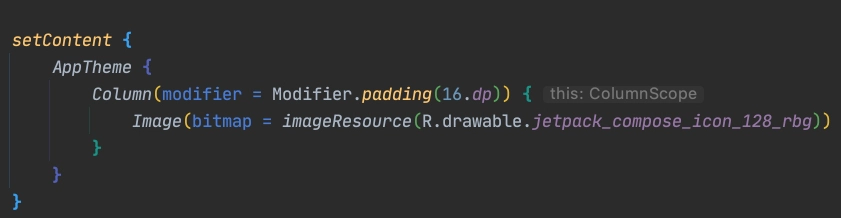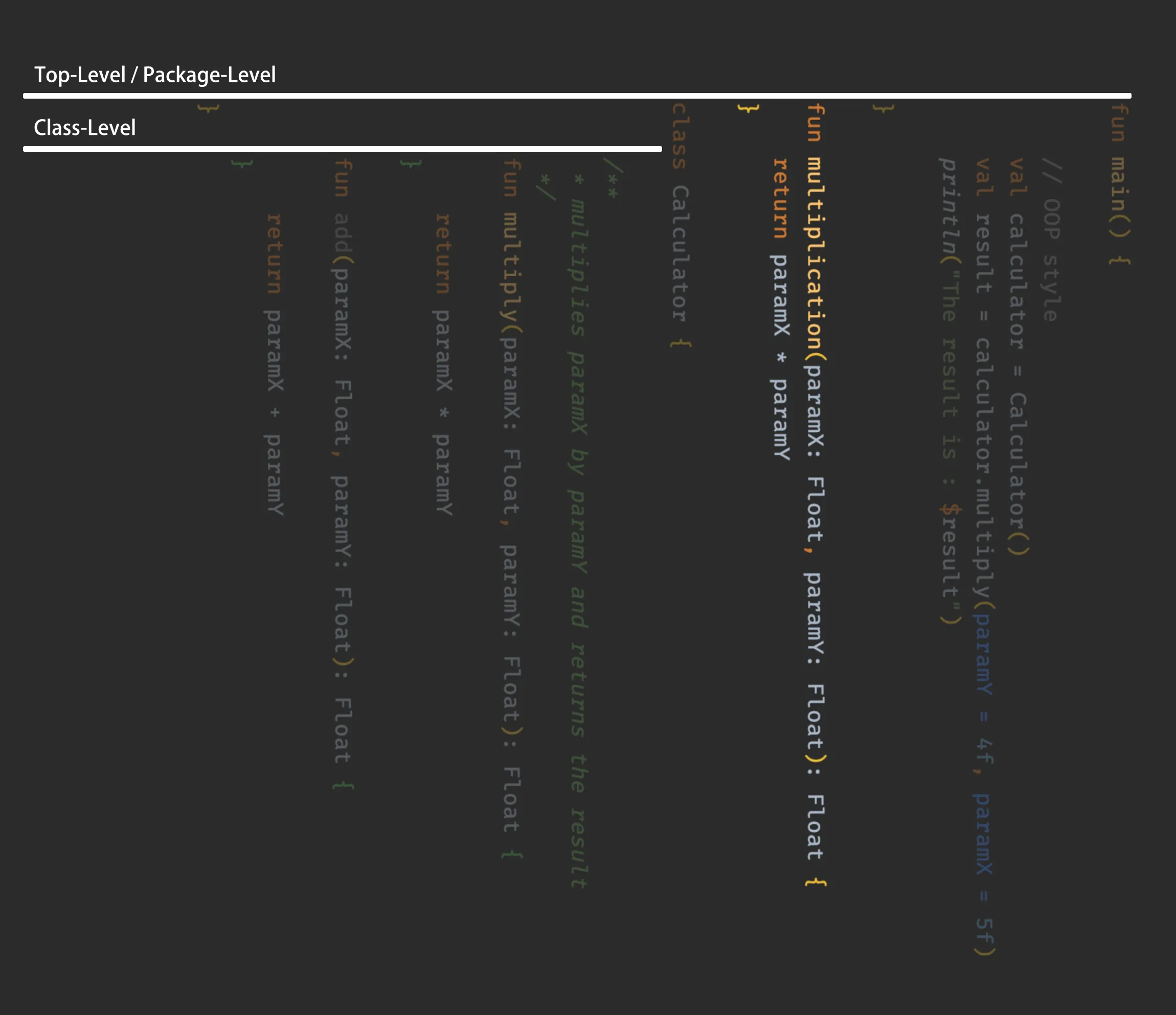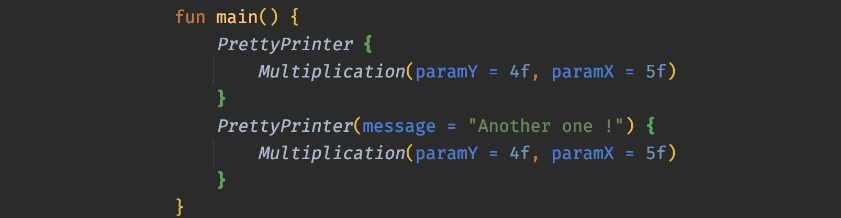Edit history of this post can be found in the blog’s Github repository.

I believe that if you are reading this article, you already know that Jetpack Compose is a new Kotlin framework for building UIs for Android applications, and also for Kotlin Desktop applications.

However, Jetpack Compose brings a syntax that may look strange for some of us - developers - especially those doing mainly Object-Oriented Programming.

Therefore, I’ll try to clarify the syntax of Jetpack Compose in this article by using:

• Basic and pure Kotlin
• Interactive code that you can edit and run without leaving this web page
• What you already know about OOP

Along the path, you may get impressed by the simplicity that hides behind some of the fancy words used to explain Jetpack Compose.

# Basic Jetpack Compose Syntax

This is how the syntax of Jetpack Compose looks like in general. At the end of the article, we will compare it with our code that we will be refactoring in a step-by-step guide.Kotlin syntax used for Jetpack Compose to show an Image

# From OOP to Function Composition

## Basic OOP

Let’s say we want to print the result of the multiplication of two floats. By using the classical approach of Object-Oriented Programming, we can write something like this in Kotlin:

You can actually run this code snippet to test it !

This Kotlin code can get more compact, but we will keep it as explicit as it can be for the sake of clarity.

The three lines of code in the `main()` function represent the usual three basic steps that we generally use in Object-Oriented Programming

• Instantiating an object
• Tell the object to do the work by invoking one if its methods
• Present the result to the user

Now, let’s find out a way to implement the same functionality but by writing the code differently.

## Getting to Top-Level

• First, we will add a function that multiplies two parameters. But we will place it outside of any class.
• Instead of giving it a verb as a name like `multiply`, we will give it a noun as a name like `multiplication`.

Check the new added lines bellow the comment `// The new style`

You can see the hidden code by clicking on the (+) signe in the code window.

By putting a function outside any class or interface in Kotlin, we are making it a top-level function. We can understand why it’s called top-level just by rotating the editor’s canvas.Why it’s called top-level function

Let’s do the same for the function that prints the result.

• Declare a new printing function as a top-level function
• Give it a noun name. Let’s call it `prettyPrinter`.

Our code will look like this:

By running this code snippet, you can verify that we still have the same result printed to the user.

## Changing your point of view

Let’s say that we don’t want to use an intermediate variable to store the result of multiplication. In our example, this means deleting the use of the variable `multiplicationResult`.

There are two ways to do it.

The first, which is classical OOP and you may already guessed it, is by deleting the parameter from `prettyPrinter` function and replacing it by a call (invocation) of the function `multiplicationResult` inside of the String message like this:

It’s true that the program will give the same result, but unfortunately it’s nowhere near the syntax of Jetpack Compose that we are looking for.

Note also that the function `prettyPrinter` now prints exclusively the result of the function `multiplication` with the specified parameters instead of printing any `Float` passed to it. Which makes the function `prettyPrinter` not that reusable !

Let’s try the second way of doing things.

By going back to our code we had this:

``````    // The new style
val multiplicationResult = multiplication(paramY = 4f, paramX = 5f)
prettyPrinter(result = multiplicationResult)
``````

Now, before deleting the variable `multiplicationResult`, we will play with its type.

The actual type `multiplicationResult` is `Float`, which is the type returned by the function `multiplication`. Therefore, we can write this :

``````    // The new style
val multiplicationResult: Float = multiplication(paramY = 4f, paramX = 5f)
prettyPrinter(result = multiplicationResult)
``````

Please look at `multiplicationResult` declaration.

The declaration means that you represent the result of the invocation of the function `multiplication` by a variable.

What about the representation of the function by itself ? Is there a way to declare it ?

Fortunately, the answer is yes, and this is how it will look like:

``````    // The new style
val operation: () -> Float = { multiplication(paramY = 4f, paramX = 5f) }
``````

You can read it as follows:

• On the left side : the variable `operation` is of type `() -> Float` which means that it’s of type function that does something and returns a Float.

• On the right side : we describe what this function actually does, which is represented by what’s inside the curly braces `{ }`. In our case, it does multiplication and returns the result as a `Float`.

Now, lets refactor our code to use this new function representation.

## Going Higher-Order

Our function `prettyPrint` was a simple function accepting a `Float` as a parameter like this

``````fun prettyPrinter(result: Float) {
println("The result is : \$result")
}
``````

In order to pass the new representation of our `multiplication` function as parameter, we need to refactor the parameter of `prettyPrinter` to look like this:

``````fun prettyPrinter(operationAsParam: () -> Float) {
println("The result is : \$operationAsParam")
}
``````

Note that :

• We changed the name of the parameter to `operationAsParam` just for clarification
• The type of the parameter has changed from `Float` to `() -> Float`

Our program will look like this after refactoring:

I’ll explain later why the result of multiplication is not displayed 😉

By doing this refactoring, a fancy expression can be used to describe the function `prettyPrinter`. We can say that it’s Higher-Order Function

Which simply means that it accepts a function as a parameter.

Now you know this, let’s keep going on with our refactoring.

## Invoking the function parameter

If you run the program where we have left, you will get this result: `Function0<java.lang.Float>` instead of printing the result of the multiplication (in our case `20.0`).

This is simply because we are trying to print the representation of the function by using directly the name of the parameter in `\$operationAsParam` instead of printing the value returned after invoking (running) the function.

To fix this, you just need to modify the String Template from `\$operationAsParam`:

``````    println("The result is : \$operationAsParam")
``````

to an invocation by adding parenthesis `()` after the name of the parameter :

``````    println("The result is : \${operationAsParam()}")
``````

This will actually replace the function parameter name with the value returned by the invocation of the function that the parameter represents.

Feel free to go back and edit the interactive code snippet ⬆️

## Being Anonymous

Thanks to our last factoring, we can now delete the intermediate variable called `operation` to go from this:

``````    val operation: () -> Float = { multiplication(paramY = 4f, paramX = 5f) }
prettyPrinter(operationAsParam = operation)
``````

to this :

``````    prettyPrinter(operationAsParam = { multiplication(paramY = 4f, paramX = 5f) } )
``````

We can simplify it by deleting the optional named parameter like this :

``````    prettyPrinter( { multiplication(paramY = 4f, paramX = 5f) } )
``````

Now we don’t have any intermediate variable 👌 and our code will run like a charm !

Let me explain something here, by deleting the intermediate variable, we deleted what identifies our function ```{ multiplication(paramY = 4f, paramX = 5f) }```. Therefore, in programming we call it an Anonymous Function !

In Kotlin, when we have a higher-order function (in our case `prettyPrinter`) which has only one parameter passed as an anonymous function expression (like in our case ```{ multiplication(paramY = 4f, paramX = 5f) }```) we can delete the parenthesis and write this:

``````    prettyPrinter { multiplication(paramY = 4f, paramX = 5f) }
// or
prettyPrinter {
multiplication(paramY = 4f, paramX = 5f)
}
``````

Feel free to edit the interactive code to test it by yourself ⬆️

By doing this, we can say that we have achieved a syntax that actually looks like the syntax of Jetpack Compose just by combining functions.

In programming this is called function composition. (Which makes sense for Jetpack Compose 😉)

## Trailing Lambda

We will add a small thing to our code to make it more Compose-alike.

Actually, the function `prettyPrinter` prints the concatenation of a hard coded message with the result, which is not really flexible. To avoid this, we will pass it as a parameter like this:

``````    fun prettyPrinter(message: String, operationAsParam: () -> Float) {
println("\$message \${operationAsParam()}")
}
``````

By doing this, we need to pass its value when calling the function like this:

By now you may ask : why would we write only one parameter inside the parenthesis (The `message` parameter) while the function has two parameters ?

The answer is that it’s a convention in Kotlin. It’s true that `prettyPrinter` has two parameters, but the last one (named `operationAsParam`) is a function. Therefore, when calling the `prettyPrinter` function, we can write the function expression passed as parameter outside the parenthesis.

This convention is called Trailing Lambda.

## The one last thing to do

• Just capitalize the names of the two functions to get `PrettyPrinter(...` and `Multiplication(...`
• Give the `message` parameter a default value to avoid writing it when calling the function each time.

You are done now, Bravo !

You can check the final code bellow and test the result

By doing these two actions you are ensuring that:

• You respect the convention used in Jetpack Compose where functions annotated with `@Composable` have capitalized names.
• You make required and optional function parameters very clear.

# The Final Result

As you can see, the final representation using only functions (function composition) gives as the same result given by the OOP style.

This is the mindset that you will need when reading or writing UI code with Jetpack Compose, it’s all about functions !# Thank you !

I hope that this article helped you mind the gap that you might encounter when reading Jetpack Compose’ code for the first time. If you feel that you have grasped the syntax, I encourage you to try to understand what Compose does behind the scenes (some of that is well explained by Leland Richardson in this talk)

Thank you for taking the time to read this article :)

Comment using Github issues to avoid cross-site trackers.

Privacy Notice : This article has one tracker integrated in the iframes provided by https://play.kotlinlang.org

Written by Oussama Hafferssas. Thanks to Sebastian Aigner for reviewing the content.